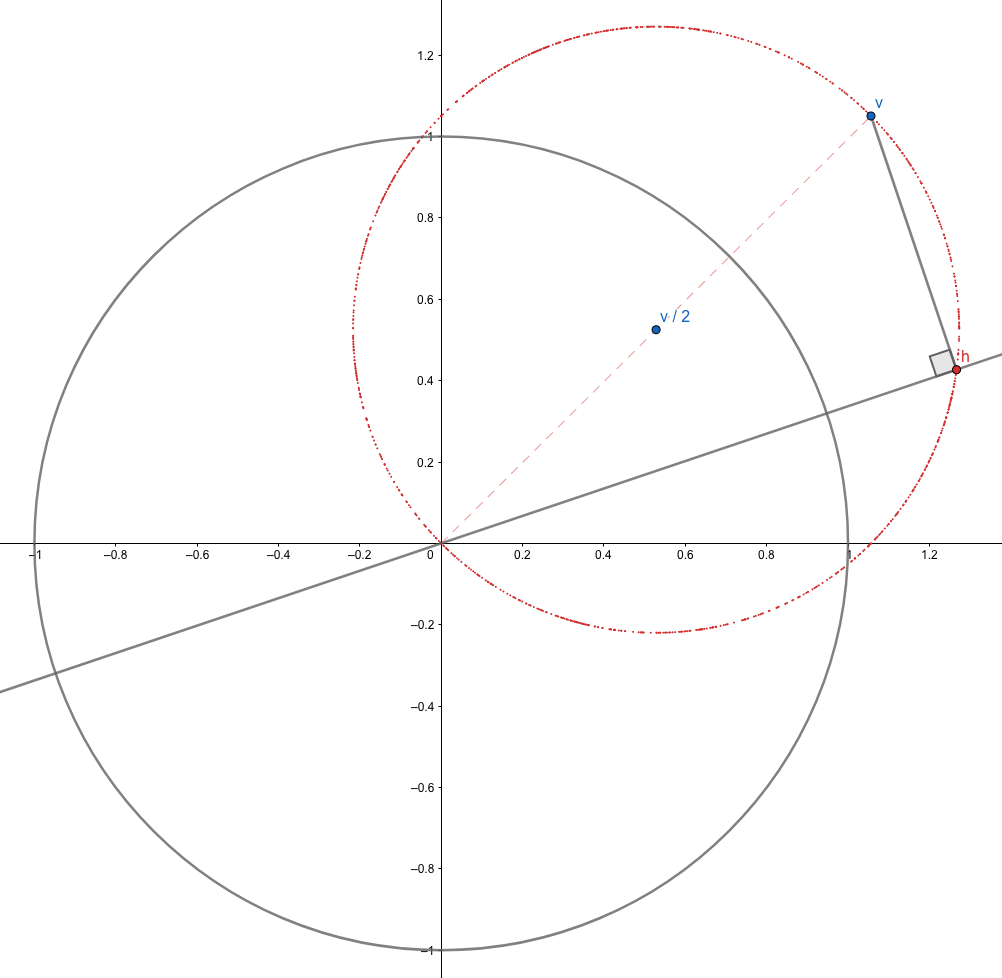# Random Projection and Its Expectation

### 2019-03-16

A couple of months ago I was asked the following question during an interview (for propriatary concerns I’m not gonna disclose the industry or name of the company): $$\newcommand{R}{\mathbb{R}} \newcommand{E}{\text{E}} \newcommand{bs}{\mathbf} \newcommand{N}{\mathbb{N}}$$

Assume $$k$$, $$n\in\N$$ and $$k < n$$. For a uniformly chosen subspace $$\R^k\subsetneq\R^n$$ we define the orthogonal projection as $$P:\R^n\mapsto\R^n$$. Find $$\E[P(\bs{v})]$$ where $$\bs{v}\in\R^n$$ is given.

It’s an interesting question and also a totally novel one to me at that time. How do we define a “uniformly” chosen subspace and its corresponding projection? What are the possible intuitions in this simple piece of question? Despite the busy schoolwork and student projects, these thoughts persist in my mind and drive me digging this question from time to time. Curiosity has been aroused and an appetite is meant to be satisfied.

# Problem Re-formulation

In order to solve this problem, we need to fully understand what’s been asked. So now we’ve got two non-negative integers $$k<n$$ and two spaces, namely $$\R^n$$ and $$\R^k$$. We know $$\R^k$$ is somehow randomly selected as a subspace of $$\R^n$$ and this randomness is uniform. For each of such selection, we can make an orthogonal projection of the given point1 $$\bs{v}$$ onto $$\R^k$$. Note here the projection is defined from $$\R^n$$ to $$\R^n$$, which means we’re not interested in the projected value on $$\R^k$$ but the projection itself. In other words, we’re focusing on the projected vector’s behavior in the same space as $$\bs{v}$$ here.

# Simple Example

The simplest example (well, it’s in fact not THE simplest as we could always project $$\bs{v}$$ onto $$\R^0$$ and the resulting expectation would be a zero vector) would be $$n=2$$ and $$k=1$$. For any given $$\bs{v}$$, we can always draw a graph as below.The random subspace in this case is illustrated by the straight gray line, which determines the projection $$P(\bs{v})=\bs{h}$$ as in the graph. We know we’re now uniformly selecting this subspace when we rotate this line centered at the origin accordingly. This means the angle between $$\bs{v}$$ and $$\bs{h}$$, denoted by $$\theta$$, is a uniform random variable on $$[0, 2\pi)$$. Further, simple geometry tells us the angle between $$\bs{v}$$ and $$\bs{h} - \bs{v}/2$$ is merely $$2\theta$$, which is therefore, also uniformly distributed on $$[0, 4\pi)$$. Now that we know $$\bs{h}$$ uniformly lies on the red circle, we conclude the expected projection, in this particular case, is $$\bs{v}/2$$.

While it gets geometrically difficult to imagine, not to mention to draw, the case of larger $$n$$ and $$k$$, this example has given us a pretty nice guess:

$\E[P(v)] = \frac{k}{n}\bs{v}.$

Can we prove it in higher dimensions and general cases?

# Rigorous Proof

Proof. Now we try to prove that our previous statement is true. For any set of orthogonal bases2 $$\bs{e}=(\bs{e}_1,\bs{e}_2,\dots,\bs{e}_n)\in\R^{n\times n}$$, we uniformly choose a subset $$(\bs{e}_{n_1}, \bs{e}_{n_2},\dots,\bs{e}_{n_k})$$ and define a subspace $$\R^k$$ on them. The projected value on any basis $$\bs{e}_j$$ is $$\bs{e}_j'\bs{v}$$ and the corresponding vector component would be $$\bs{e}_j\bs{e}_j'\bs{v}$$. Therefore, the orthogonal projection of $$\bs{v}$$ is given by

$P(\bs{v}) = \sum_{j=1}^{k}\bs{e}_{n_j}\bs{e}_{n_j}'\bs{v} = \bs{eDe'v} \in \R^n$

where we define the random matrix $$\bs{D}$$ to be a diagonal matrix with $$k$$ ones and $$(n-k)$$ zeros on its diagonal. The diagonal entries are not independent, but the expectation of each entry would be the same, namely $$k/n$$. The expectation of the projection, therefore, is

$\E[P(\bs{v})] = \E[\bs{eDe'v}] = \E\{\E[\bs{eDe'v}\mid \bs{e}]\} = \E\{\bs{e}\E[\bs{D}]\bs{e'v}\} = \frac{k}{n}\E[\bs{ee'}]\bs{v}.$

where we used the tower rule3. Now, notice for any $$\bs{e}$$ it always holds that $$\bs{e'e}=\bs{I}$$, we have

$(\bs{ee'})^2 = \bs{ee'ee'} = \bs{e(e'e)e'} = \bs{eIe'} = \bs{ee'} \Rightarrow \bs{ee'} = \bs{I}$

and thus we may finally conclude

$\E[P(\bs{v})] = \frac{k}{n}\E[\bs{ee'}]\bs{v} = \frac{k}{n}\bs{v}$

which exactly coincides with our previous guess.Q.E.D.

# Intuitive Implications

TBD. May concern dimensional reduction, etc.

1. Points and vectors are denoted in bold and defined as column vectors unless otherwise stated. ↩︎
2. When we say $$\bs{a}=(a_1,a_2,\dots,a_n)$$, we’re talking about a row vector of $$n$$ entries. ↩︎
3. For any r.v. $$X$$, we claim $$\E[X] = \E\{\E[X\mid Y]\}$$. Also known as the law of total expectation. ↩︎